Atlanta Real Food is run by the Atlanta area chapter leaders of The Weston A. Price Foundation. Here you will find the latest news from local farmers, get information on how to properly prepare real foods, and stay up to date on local events.

Friday, November 23, 2018

We are gratefulLearn to be grateful for what you already have, while you pursue all that you want. -Jim Rohn

 table div table+table+table+table div table{width:100%;padding:0}table div table+table+table+table div table img{width:96.23%;padding:0;float:none}table div table+table+table+table div table td{width:100%;padding:0 1.88% 18px}/* styles *//* styles */ Now is the time that many of think about all the things we have to be grateful for ... family, friends, a home, jobs...

But did you know that taking time to be grateful everyday has its rewards?

 table div table+table+table+table+table+table+table+table div table{width:100%;padding:0}table div table+table+table+table+table+table+table+table div table img{width:96.23%;padding:0;float:none}table div table+table+table+table+table+table+table+table div table td{width:100%;padding:0 1.88% 18px}/* styles */At Alegre Farm, We, Too, Are Grateful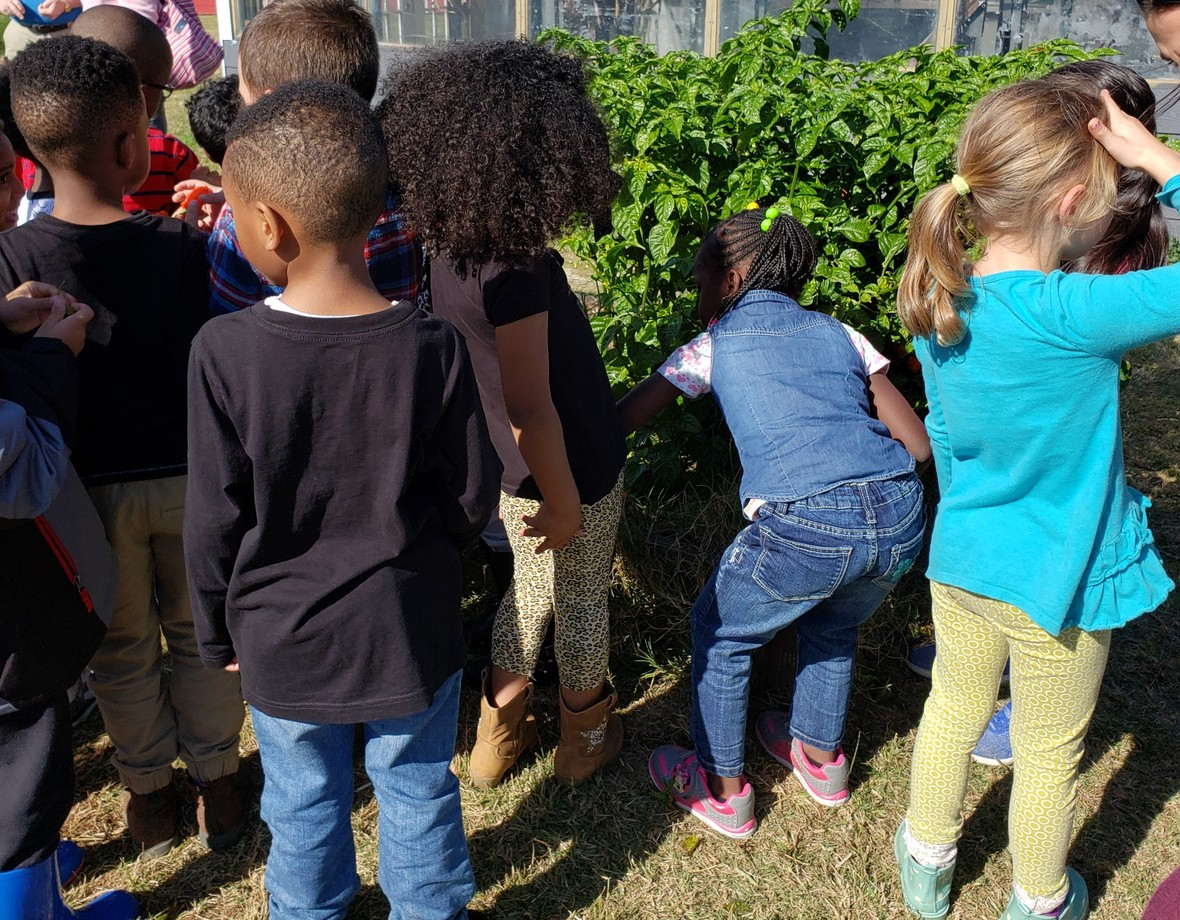To share with our communities and future generations

that food doesn't grow in the supermarket. That worms have an important role in making healthy soil for our plants. That bees aren't just insects that sting, but share a vital role in our food production. That hens lay eggs and milk comes from goats, too!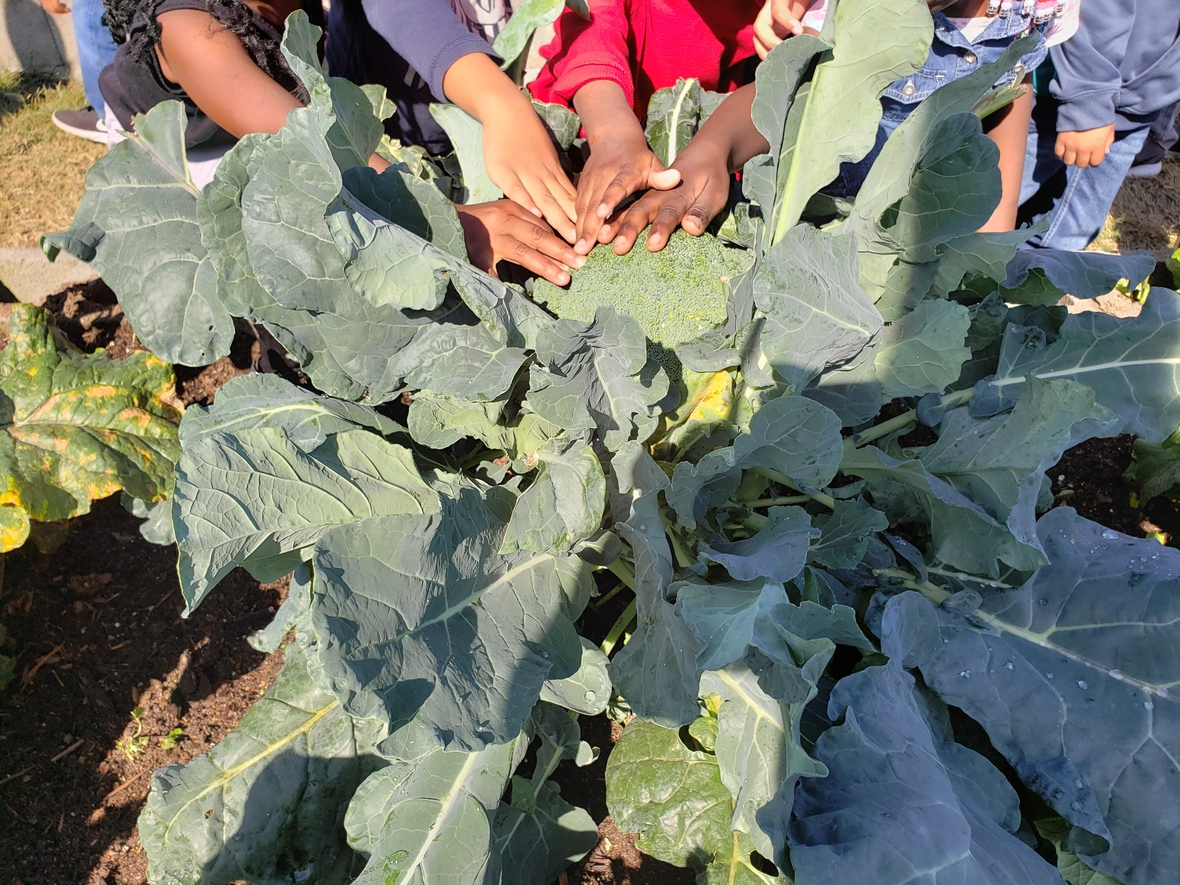We are grateful everyday to have the privilege to share with you...

It's exciting to see that both children and adults are fascinated by our garden and orchard! Do you know how broccoli and brussel sprouts grow? Have you smelled the different herbs of mint, oregano, and rosemary? These are things that delight us, and we are thankful and hope to continue connecting with our communities and future generations.
A sincere thank you for all your support from all of us at Alegre Farm

 table div table+table+table+table+table+table+table+table+table+table+table+table div table{width:100%;padding:0}table div table+table+table+table+table+table+table+table+table+table+table+table div table img{width:96.23%;padding:0;float:none}table div table+table+table+table+table+table+table+table+table+table+table+table div table td{width:100%;padding:0 1.88% 18px}/* styles */Bring the Family to Farm Adventure Day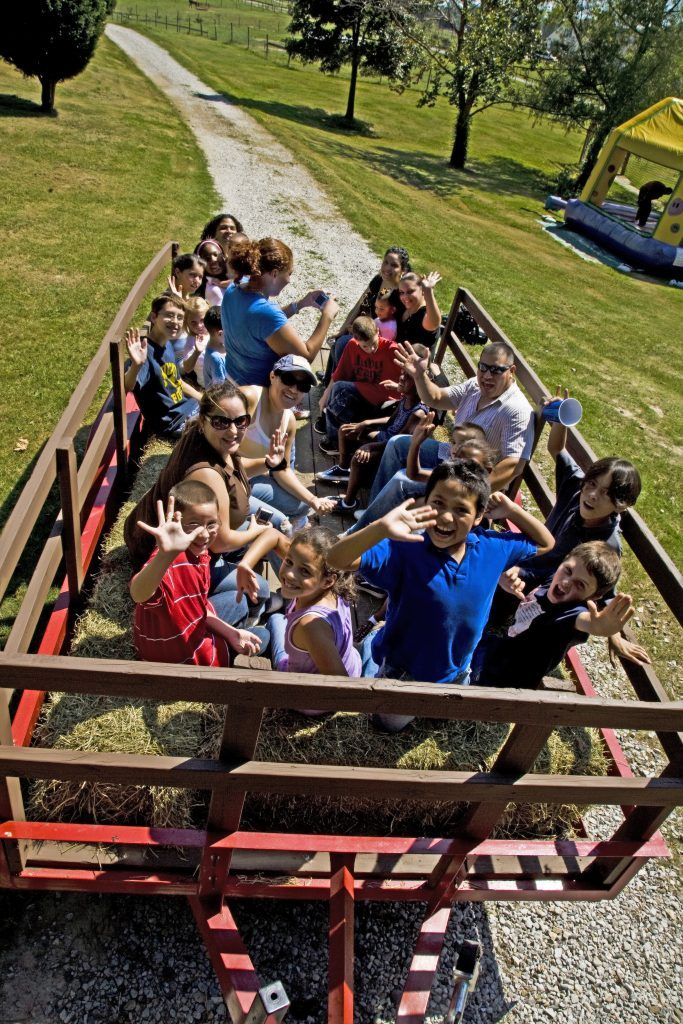Friday, December 21st

Join us this winter break as we open the farm to the public to enjoy our educational stations. Get early bird pricing. Tickets limited!

 table div table+table+table+table+table+table+table+table+table+table+table+table+table+table+table+table div table{width:100%;padding:0}table div table+table+table+table+table+table+table+table+table+table+table+table+table+table+table+table div table img{width:96.23%;padding:0;float:none}table div table+table+table+table+table+table+table+table+table+table+table+table+table+table+table+table div table td{width:100%;padding:0 1.88% 18px}/* styles */Book your photo shoot with Santa... Limited Spots!table div table+table+table+table+table+table+table+table+table+table+table+table+table+table+table+table+table+table+table div table{width:100%;padding:0}table div table+table+table+table+table+table+table+table+table+table+table+table+table+table+table+table+table+table+table div table img{width:96.23%;padding:0;float:none}table div table+table+table+table+table+table+table+table+table+table+table+table+table+table+table+table+table+table+table div table td{width:100%;padding:0 1.88% 18px}/* styles */We're hiringJob Opening

We are currently seeking a full-time, highly-organized administrative assistant to join the Alegre Farm team. Those who wish to apply must have knowledge of marketing, event planning, product launches, and leadership skills. We ask that candidates are able to work with little direction and are innovative in their thinking.

 table div table+table+table+table+table+table+table+table+table+table+table+table+table+table+table+table+table+table+table+table+table+table div table{width:100%;padding:0}table div table+table+table+table+table+table+table+table+table+table+table+table+table+table+table+table+table+table+table+table+table+table div table img{width:96.23%;padding:0;float:none}table div table+table+table+table+table+table+table+table+table+table+table+table+table+table+table+table+table+table+table+table+table+table div table td{width:100%;padding:0 1.88% 18px}/* styles */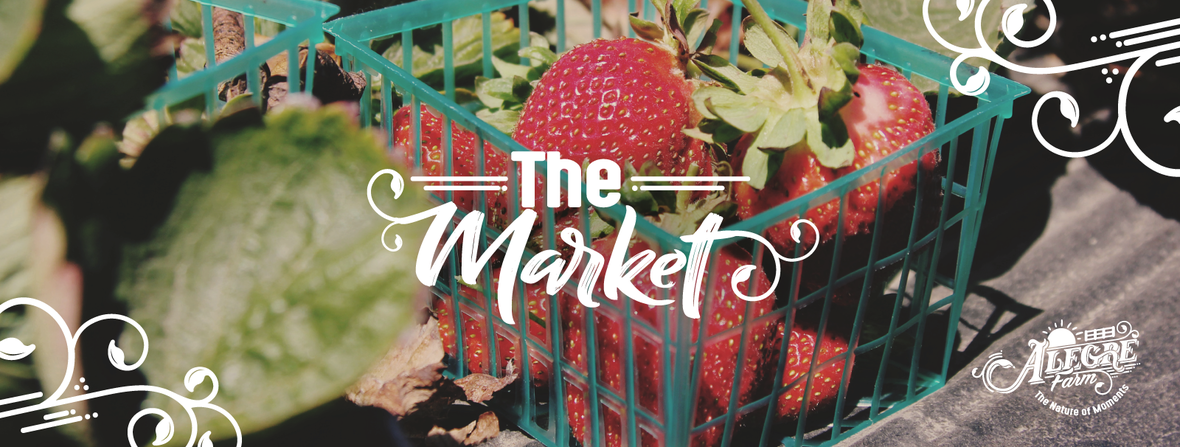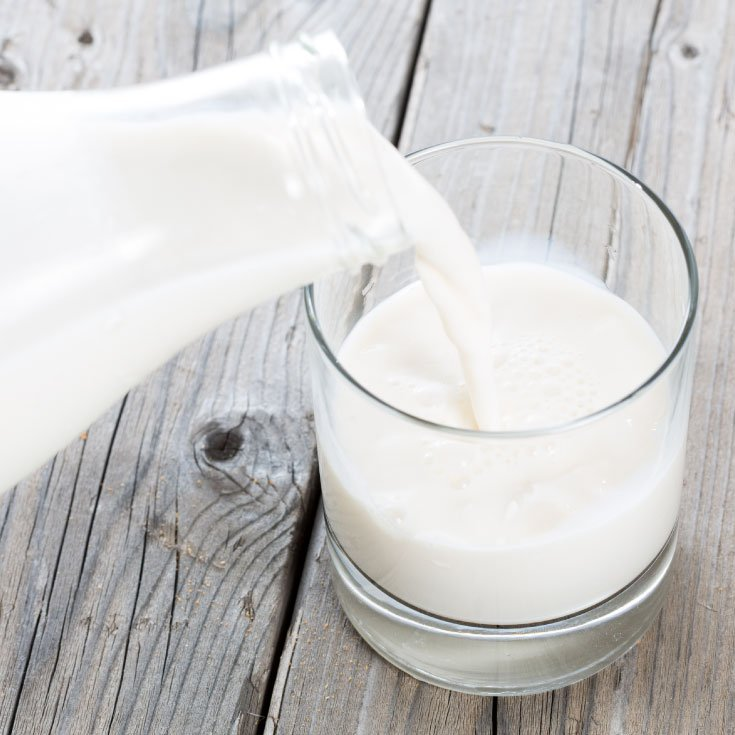raw milk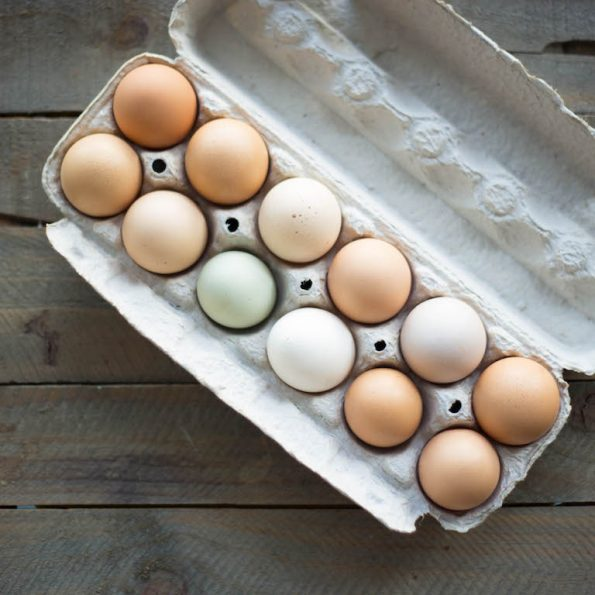fresh eggs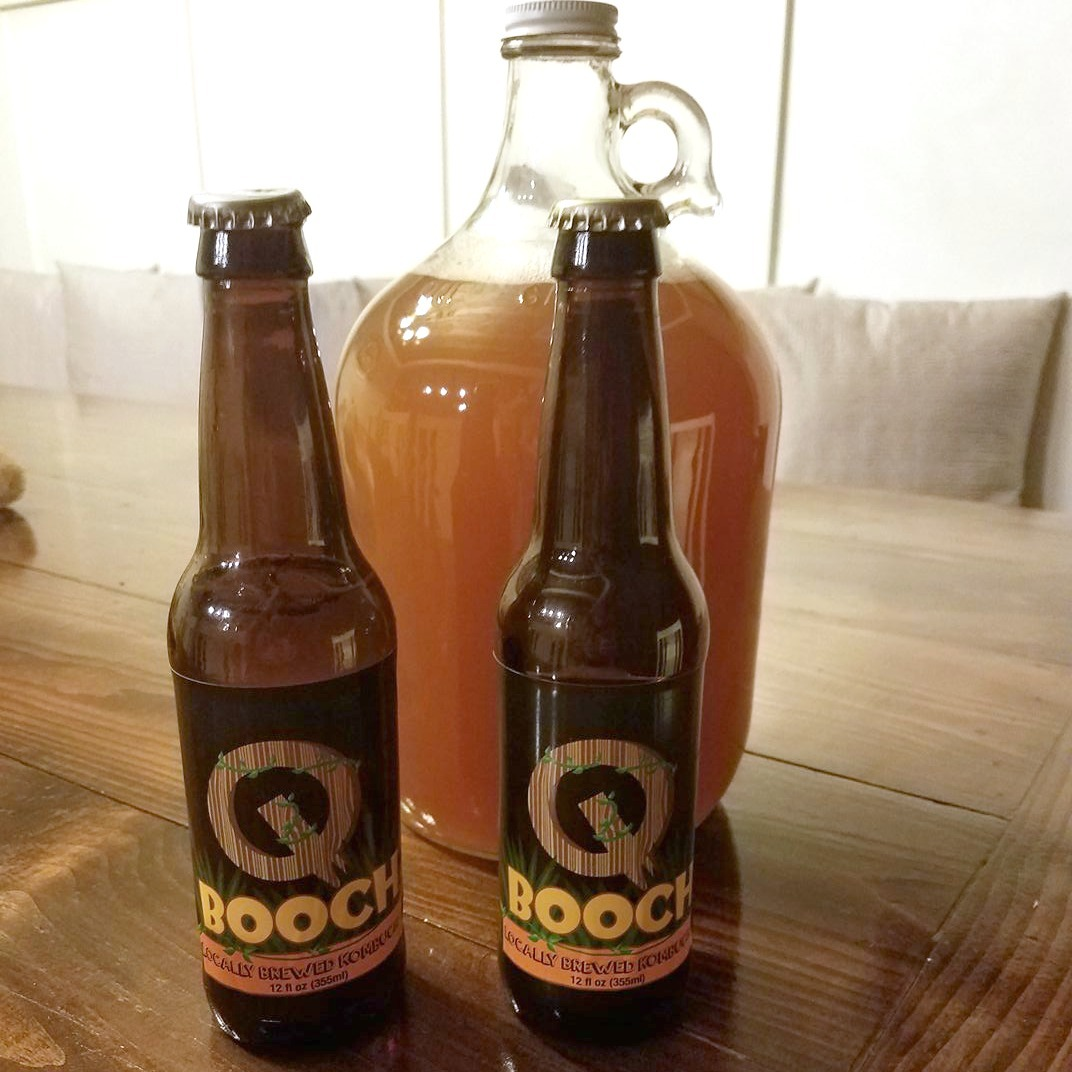kombucha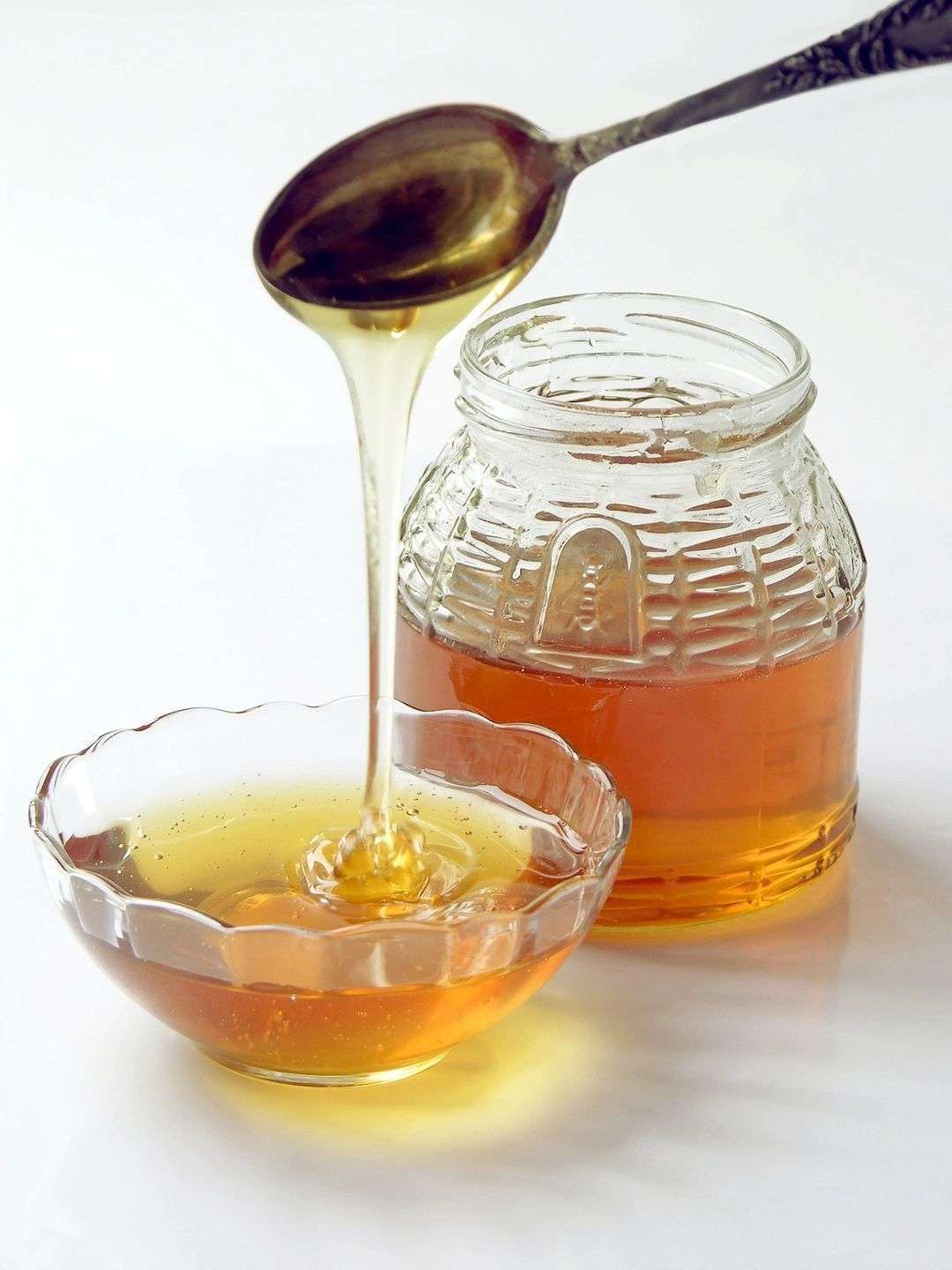raw honey
 table div table+table+table+table+table+table+table+table+table+table+table+table+table+table+table+table+table+table+table+table+table+table+table+table+table+table+table+table div table{width:100%;padding:0}table div table+table+table+table+table+table+table+table+table+table+table+table+table+table+table+table+table+table+table+table+table+table+table+table+table+table+table+table div table img{width:96.23%;padding:0;float:none}table div table+table+table+table+table+table+table+table+table+table+table+table+table+table+table+table+table+table+table+table+table+table+table+table+table+table+table+table div table td{width:100%;padding:0 1.88% 18px}/* styles */